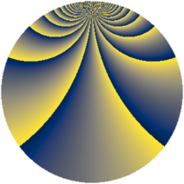# Properties

 Label 2001.2.yLevel $2001$ Weight $2$ Character orbit 2001.y Rep. character $\chi_{2001}(202,\cdot)$ Character field $\Q(\zeta_{22})$ Dimension $1200$ Sturm bound $480$

# Related objects

## Defining parameters

 Level: $$N$$ $$=$$ $$2001 = 3 \cdot 23 \cdot 29$$ Weight: $$k$$ $$=$$ $$2$$ Character orbit: $$[\chi]$$ $$=$$ 2001.y (of order $$22$$ and degree $$10$$) Character conductor: $$\operatorname{cond}(\chi)$$ $$=$$ $$667$$ Character field: $$\Q(\zeta_{22})$$ Sturm bound: $$480$$

## Dimensions

The following table gives the dimensions of various subspaces of $$M_{2}(2001, [\chi])$$.

Total New Old
Modular forms 2440 1200 1240
Cusp forms 2360 1200 1160
Eisenstein series 80 0 80

## Trace form

 $$1200q + 112q^{4} + 8q^{7} + 120q^{9} + O(q^{10})$$ $$1200q + 112q^{4} + 8q^{7} + 120q^{9} + 8q^{13} - 200q^{16} + 80q^{22} - 84q^{23} - 40q^{25} + 22q^{29} - 24q^{30} - 16q^{33} + 16q^{34} + 24q^{35} - 156q^{36} + 228q^{38} + 8q^{42} - 144q^{49} + 56q^{51} + 8q^{52} - 16q^{53} - 8q^{57} + 10q^{58} + 32q^{59} + 72q^{62} - 8q^{63} + 48q^{64} - 16q^{65} + 52q^{67} - 48q^{71} + 28q^{74} + 16q^{78} - 348q^{80} - 120q^{81} - 88q^{82} - 152q^{83} - 108q^{86} + 24q^{87} + 352q^{88} + 256q^{91} + 12q^{92} - 32q^{93} + 48q^{94} + O(q^{100})$$

## Decomposition of $$S_{2}^{\mathrm{new}}(2001, [\chi])$$ into newform subspaces

The newforms in this space have not yet been added to the LMFDB.

## Decomposition of $$S_{2}^{\mathrm{old}}(2001, [\chi])$$ into lower level spaces

$$S_{2}^{\mathrm{old}}(2001, [\chi]) \cong$$ $$S_{2}^{\mathrm{new}}(667, [\chi])$$$$^{\oplus 2}$$